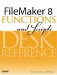# IsValidExpression()

Category: Logical

 Syntax: IsValidExpression ( expression )

Parameters:

expressionA text string containing a calculation expression, or a field or expression that returns a text string that contains a calculation expression.

Data type returned: Number

Description:

Returns 1 (true) if the expression syntax is correct. Returns 0 (False) if the expression has a syntax error.

The IsValidExpression() function is often used in conjunction with the Evaluate() function to ensure that Evaluate() is passed a valid expression. For instance, if users are allowed to enter a formula into a field called myFormula, and you want to have another field express the results of that formula, you could use the following:

``` If (IsValidExpression (myFormula); Evaluate (myFormula); "Invalid formula: "
& TextColor (myFormula; RBG (255; 0; 0)))
```

An expression is considered invalid if it contains syntax errors or if any of the referenced fields cannot be found. Errors that might occur only during execution of the expression, such as record access restrictions, are not detected by the IsValidExpression() formula.

Examples:

Function

Results

IsValidExpression ("Length (SideA)")

Returns 1 (true) as long as there is, in fact, a field named SideA.

IsValidExpression ("Middle (myField; 1)")

Returns 0 (False) because the Middle function requires three parameters to be considered valid syntax.

IsValidExpression (myFormula)

Returns 1 (true) if the contents of myFormula would be considered a valid calculation expression.FileMaker 8 Functions and Scripts Desk Reference
ISBN: 0789735113
EAN: 2147483647
Year: 2004
Pages: 352